Year 7 Interactive Maths - Second Edition

## Decimals

John Napier, a Scottish baron, introduced the decimal number system in 1619 A.D.  The decimal number system is easier to use in everyday life than fractions as it is a base 10 system.

Decimal numbers are commonly used in areas such as finance, medicine and construction when measuring units such as currency, mass and distance.  It is useful to know decimals thoroughly, as they are involved in many aspects of daily life.

## Place Value

Recall that a whole number such as 73 486 has 5 digits and the place value table for the whole number is as follows: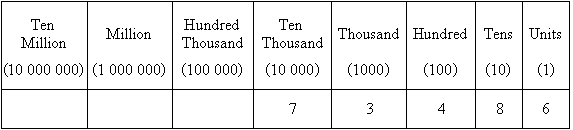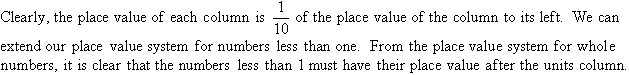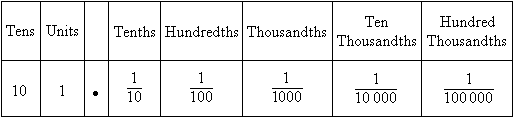In expanded fractional form, 72.649 is written as: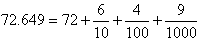So, 72.649 has seven tens, two units, six tenths, four hundredths and nine thousandths.

We find from the place value table of 72.649 that:

• The digit 6 is in the first place after the decimal point.  So, it represents: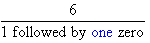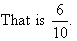• The digit 4 is in the second place after the decimal point.  So, it represents: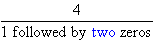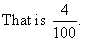• The digit 9 is in the third place after the decimal point.  So, it represents: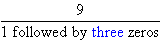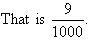###### In general:
To express the decimal place value of a number in fractional form, the position of the digit in the number tells us how many zeros are to be added after the 1 in the denominator.

For example, 3 in the decimal number 94.19547632 is in the 7th place after the decimal point.  So, it represents: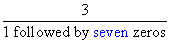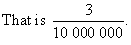#### Example 1

Give the place value of 8 in the following decimal numbers:

a.  0.856
b.  0.384
c.  0.4386
d.  0.16982

##### Solution: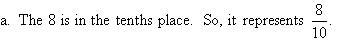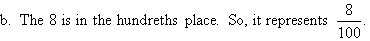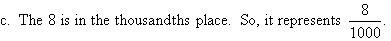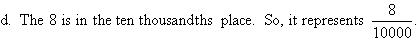#### Example 2

Write each of the following as a decimal number: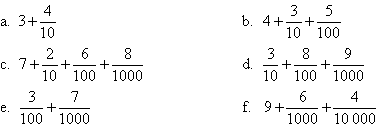##### Solution: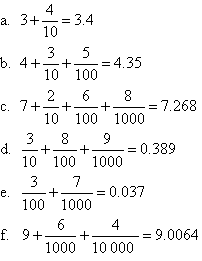#### Example 3

Write each of the following numbers in expanded fractional form: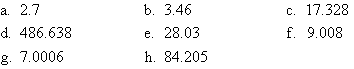##### Solution: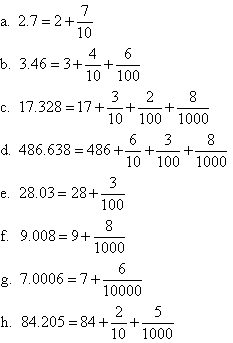#### Example 4

Write each of the following decimal numbers in words: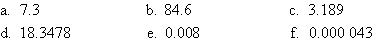##### Solution:

a.  7.3 represents seven units and three tenths.
b.  84.6 represents eight tens, four units and six tenths.
c.  3.189 represents three units, one tenth, eight hundredths and nine thousandths.
d.  18.3478 represents one ten, eight units, three tenths, four hundredths, seven thousandths and eight ten-thousandths.
e.  0.008 represents eight thousandths.
f.   0.000 043 represents four hundred-thousandths and three millionths.

###### Key Terms

Copyright © 2000-2019 mathsteacher.com Pty Ltd.  All rights reserved.
Australian Business Number 53 056 217 611

Copyright instructions for educational institutions

Please read the Terms and Conditions of Use of this Website and our Privacy and Other Policies.
If you experience difficulties when using this Website, tell us through the feedback form or by phoning the contact telephone number.